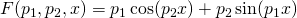## Simple nonlinear least squares curve fitting in Maple

December 6th, 2013 | Categories: Maple, math software | Tags:

A question I get asked a lot is ‘How can I do nonlinear least squares curve fitting in X?’ where X might be MATLAB, Mathematica or a whole host of alternatives.  Since this is such a common query, I thought I’d write up how to do it for a very simple problem in several systems that I’m interested in

This is the Maple version. For other versions,see the list below

The problem

xdata = -2,-1.64,-1.33,-0.7,0,0.45,1.2,1.64,2.32,2.9
ydata = 0.699369,0.700462,0.695354,1.03905,1.97389,2.41143,1.91091,0.919576,-0.730975,-1.42001

and you’d like to fit the functionusing nonlinear least squares.  You’re starting guesses for the parameters are p1=1 and P2=0.2

For now, we are primarily interested in the following results:

• The fit parameters
• Sum of squared residuals

Future updates of these posts will show how to get other results such as confidence intervals. Let me know what you are most interested in.

Solution in Maple

Maple’s user interface is quite user friendly and it uses non-linear optimization routines from The Numerical Algorithms Group under the hood. Here’s the code to get the parameter values and sum of squares of residuals

with(Statistics):

xdata := Vector([-2, -1.64, -1.33, -.7, 0, .45, 1.2, 1.64, 2.32, 2.9], datatype = float):
ydata := Vector([.699369, .700462, .695354, 1.03905, 1.97389, 2.41143, 1.91091, .919576,
-.730975, -1.42001], datatype = float):
NonlinearFit(p1*cos(p2*x)+p2*sin(p1*x), xdata, ydata, x, initialvalues = [p1 = 1, p2 = .2],
output = [parametervalues, residualsumofsquares])

which gives the result

[[p1 = 1.88185090465902, p2 = 0.700229557992540], 0.05381269642]

Various other outputs can be specified in the output vector such as:

• solutionmodule
• degreesoffreedom
• leastsquaresfunction
• parametervalues
• parametervector
• residuals
• residualmeansquare
• residualstandarddeviation
• residualsumofsquares.

The meanings of the above are mostly obvious.  In those cases where they aren’t, you can look them up in the documentation link below.

Further documentation

The full documentation for Maple’s NonlinearFit command is at http://www.maplesoft.com/support/help/Maple/view.aspx?path=Statistics%2fNonlinearFit

Notes

I used Maple 17.02 on 64bit Windows to run the code in this post.

1. Hi Mike,

Just a quick heads up – if you’re entering the data manually, you might as well save yourself some typing and keep the definitions of xdata and ydata as lists:

xdata := [-2, -1.64, -1.33, -.7, 0, .45, 1.2, 1.64, 2.32, 2.9]:
ydata := [.699369, .700462, .695354, 1.03905, 1.97389, 2.41143, 1.91091, .919576, -.730975, -1.42001]:

They get converted to float Vectors internally anyway, so there’s no difference in the actual computation. Now if you do a longer chain of computations it may be a good idea to do this conversion just once, to prevent doing the conversion multiple times (if your data is big enough that it’s worth it to think about this).

Another suggestion: the Fit command (also in the Statistics package) decides for itself if nonlinear techniques are necessary, and calls LinearFit if possible and NonlinearFit if necessary. It’s not always obvious to users that the expression you’re fitting needs to be linear only in the *parameters* and not in the independent variable(s), so sometimes this enables the computationally more straightforward linear methods where the user would otherwise have selected nonlinear methods. Of course your premise here was that the user knew they wanted nonlinear fitting, so NonlinearFit is actually a good choice here.

Thanks!

Erik Postma
Maplesoft.